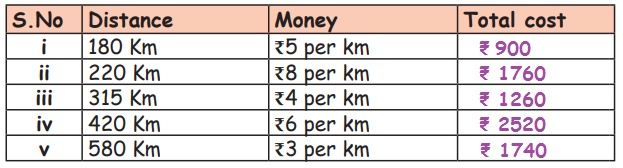Home | | Maths 5th Std | Exercise 5.3 (Relation between Distance and Money)

# Exercise 5.3 (Relation between Distance and Money)

Text Book Back Exercises Questions with Answers, Solution : 5th Maths : Term 2 Unit 5 : Interconcept : Exercise 5.3 (Relation between Distance and Money)

Exercise 5.3i. Cost per kilometer = ₹ 5

Cost for 180km = ₹5 × 180=₹900

Cost for 180km = ₹ 900

ii. Cost per kilometer = ₹ 8

Cist for 220km = ₹8 × 220 = ₹1760

Cost for 220km = ₹1760

iii. Cost per kilometer = ₹4

Cost for 315km = ₹ 4 × 315 = ₹1260

Cost for 315km = ₹ 1260

iv. Cost per kilometer = ₹6

Cost for 420km = ₹6 × 420 = ₹2520

Cost for 420km = ₹ 2520

v. Cost per kilometer = ₹3

Cost for 580km = ₹3 × 580 = ₹1740

Cost for 580km = ₹1740

2. Sneha spent ₹ 7 per Km for a trip and she travelled 850km. How much is the total cost of the trip?

Sneha spent money per km = ₹ 7

Sneha spent money for 850 km = ₹ 7 × 850 = ₹5950

Total cost of the trip = ₹ 5950

3. Prabhu spent ₹ 9per Km for a trip and he travelled 580km. How much is the total cost of the trip?

Prabhu spent money per km = ₹ 9

Prabhu spent money for 580 km = ₹ 9×580 = ₹5220

Total cost of the trip = ₹ 5220

Exercise 5.3

1. i) ₹ 900 ii) ₹ 1760 iii) ₹ 1260 iv) ₹ 2520 v) ₹ 1740

2. ₹5950

3. ₹5220

Tags : Interconcept | Term 2 Chapter 5 | 5th Maths , 5th Maths : Term 2 Unit 5 : Interconcept
Study Material, Lecturing Notes, Assignment, Reference, Wiki description explanation, brief detail
5th Maths : Term 2 Unit 5 : Interconcept : Exercise 5.3 (Relation between Distance and Money) | Interconcept | Term 2 Chapter 5 | 5th Maths